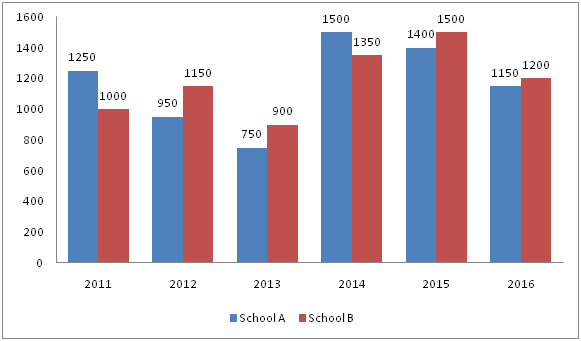# IBPS PO Prelims Quantitative Aptitude Questions 2019 (Day-1)

Dear Aspirants, Our IBPS Guide team is providing new series of Quantitative Aptitude Questions for IBPS PO Prelims 2019 so the aspirants can practice it on a daily basis. These questions are framed by our skilled experts after understanding your needs thoroughly. Aspirants can practice these new series questions daily to familiarize with the exact exam pattern and make your preparation effective.

Check here for IBPS PO Prelims Mock Test 2019

##### Check here for IBPS PO  Mock Test 2019
[WpProQuiz 6836]

Directions (Q. 1 – 5): Find the wrong term in the following number series?

1) 172, 200, 263, 388, 604, 947, 1459

a) 263

b) 388

c) 200

d) 604

e) 947

2) 34, 32, 62, 184, 732, 3668

a) 32

b) 3668

c) 184

d) 732

e) 62

3) 567, 579, 607, 649, 711, 797

a) 607

b) 579

c) 711

d) 797

e) 649

4) 225, 223, 111, 36, 8

a) 223

b) 111

c) 36

d) 225

e) 8

5) 21, 10, 11, 16.5, 32, 77.5, 235.5

a) 10

b) 38

c) 97.5

d) 295.5

e) 5

Directions (Q. 6 – 10): Study the following information carefully and answer the given questions?

The following bar graph shows the total number of students appearing for final examination from 2 different schools over the years.6) Total number of students appeared for final examination from School A in the year 2011 and 2015 together is what percentage of total number of students appeared for final examination from School B in the year 2012 and 2014 together?

a) 85 %

b) 92 %

c) 106 %

d) 124 %

e) None of these

7) Find the difference between the total number of students appeared for final examination from School A to that of School B in all the given years?

a) 100

b) 150

c) 50

d) 200

e) None of these

8) If the percentage of total number of students passed in School A and B in 2016 is 82 % and 91 % respectively, then ratio between the total number of students appeared for final examination from both the schools in 2016 to that of total number of students passed from both the schools in 2016?

a) 157 : 189

b) 28 : 45

c) 11 : 5

d) 470 : 407

e) None of these

9) If the ratio of total number of male to that of female students appeared for final examination from School A in 2011 and 2013 is 13 : 12 and 7 : 8 respectively, then find the average number of female students appeared for final examination from School A in 2011 and 2013?

a) 650

b) 500

c) 525

d) 675

e) None of these

10) Find the average number of students appeared from School B in the year 2011, 2013, 2015 and 2016 together?

a) 2200

b) 2100

c) 2050

d) 2250

e) None of these

Direction (1-5) :

The correct series is,

172, 199, 263, 388, 604, 947, 1459

The pattern is, +33, +43, +53, +63, +73, +83,..

The wrong term is, 200

The correct series is,

34, 32, 62, 184, 734, 3668

The pattern is, *1 – 2, *2 – 2, *3 – 2, *4 – 2, *5 – 2,..

The wrong term is, 732

The correct series is,

567,            581,            607,            649,            711,            797

14               26               42               62               86

12               16               20               24

The difference of difference is, 12, 16, 20, 24,…

The wrong term is, 579

The correct series is,

225, 224, 111, 36, 8

The pattern is,

= > (225 – 1)/1 = 224, (224 – 2)/2 = 111, (111 – 3)/3 = 36, (36 – 4)/4 = 8

The wrong term is, 223

The correct series is,

21, 10, 11, 15, 32, 77.5, 235.5

The pattern is, *0.5 – 0.5, *1 + 1, *1.5 – 1.5, *2 + 2, *2.5 – 2.5, *3 + 3,….

The wrong term is, 16.5

Direction (6-10) :

Total number of students appeared for final examination from School A in the year 2011 and 2015 together

= > 1250 + 1400 = 2650

Total number of students appeared for final examination from School B in the year 2012 and 2014 together

= > 1150 + 1350 = 2500

Required % = (2650 / 2500) * 100 = 106 %

The total number of students appeared for final examination from School A in all the given years

= > 1250 + 950 + 750 + 1500 + 1400 + 1150 = 7000

The total number of students appeared for final examination from School B in all the given years

= > 1000 + 1150 + 900 + 1350 + 1500 + 1200 = 7100

Required difference = 7100 – 7000 = 100

The total number of students appeared for final examination from both the schools in 2016

= > 1150 + 1200 = 2350

The total number of students passed from both the schools in 2016

= > 1150 * (82/100) + 1200 * (91/100)

= > 943 + 1092 = 2035

Required ratio = 2350 : 2035 = 470 : 407

The ratio of total number of male to that of female students appeared for final examination from School A in 2011 = 13 : 12

The total number of female students appeared for final examination from School A in 2011

= > 1250 * (12/25) = 600

The ratio of total number of male to that of female students appeared for final examination from School A in 2013 = 7 : 8

The total number of female students appeared for final examination from School A in 2013

= > 750 * (8/15) = 400

The average number of female students appeared for final examination from School A in 2011 and 2013

= > (600 + 400) / 2 = 1000 / 2 = 500